# Electronics and Communication Engineering - Analog Electronics

36.

Which of the following parameters of CE circuit has the unit of ohms?

 A. hie B. hre C. hfe D. hoe

Explanation:

No answer description available for this question. Let us discuss.

37.

In the op-amp circuit shown in figure the current through load resistance RL is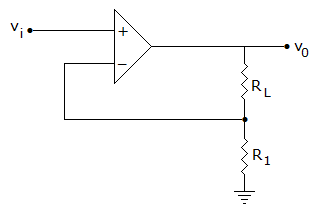A.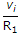B.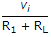C.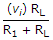D.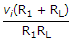Explanation:

No answer description available for this question. Let us discuss.

38.

Whenever a JFET operates above pinch-off voltage

 A. depletion regions become smaller B. drain current increase steeply C. drain current starts decreasing D. drain current remains nearly constant

Explanation:

No answer description available for this question. Let us discuss.

39.

A Weinbridge oscillator is a

 A. VHF oscillator B. RF oscillator C. microwave oscillator D. audio frequency oscillator

Explanation:

No answer description available for this question. Let us discuss.

40.

RC network shown in the given figure can provide a maximum theoretical phase shift of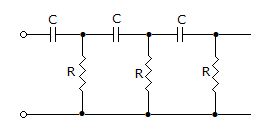A. 90° B. 180° C. 270° D. 360°